## SDR in ORBIT: Spectrum Sensing

### Introduction

Spectrum sensing is the systematic scanning of the available radio frequency spectrum for areas of high usage. Our project involves the use of Universal Software Radio Peripherals (USRPs) in ORBIT to detect which frequencies are in use at any given time. Using WINLAB's Wiserd framework and MATLAB/Octave for signal processing, we aim to detect with optimal speed and efficiency the frequency of any signal transmitted from an unknown source. Accomplishing this goal will help to identify available bands in the frequency spectrum, giving opportunities for more efficient usage.

### TeamChristina BaakliniElectrical and Computer EngineeringRutgers University Michael CollinsElectrical and Computer EngineeringRutgers University Nick CooperHigh School StudentMontgomery High School Nicole DiLeoElectrical and Computer EngineeringRutgers University

Project guided by Wade Trappe, Dola Saha, and Ivan Seskar.

### Objectives

• Knowledge of basic Digital Signal Processing concepts
• Knowledge of filter design
• Utilization of ORBIT
• Writing/editing scripts
• Saving from console to local devices
• Running experiments on the grid
• Utilization of MATLAB to process signals
• Fast Fourier Transform (FFT)
• Inverse Fast Fourier Transform (IFFT)
• Design of Signal Processing and Data Visualization Tools
• Transform IQ samples to frequency domain
• Analyze data and identify any transmitted frequencies
• Generate waterfall plots, power vs. frequency plots, and other visuals
• Real-Time Application
• Display visuals in real-time
• User interface for changing receiver carrier frequency, sampling rate, etc.

### Weekly Progress

Weeks 1-2 (Presentation)

• Formation of project group.
• Development of project goals.
• Preliminary research on software-defined radio.

Week 3 (Presentation)

• Research on analog-to-digital conversion.
• Familiarization with Fast Fourier Transform (FFT) in MATLAB.
• USRP Tutorials using ORBIT.

Week 4 (Presentation)

• Research on filter design.
• Writing/Modification of OEDL scripts. See Experiment 1.
• Development of a MATLAB script for signal visualization.

Week 5 (Presentation)

• Introduction of FPGA into the project.
• Use of OML to gather raw IQ samples from USRPs.
• Development of a MATLAB spectrogram script.

Week 6 (Presentation)

• FPGA Programming Using VHDL.
• Experimentation on the ORBIT Grid. See Experiment 2.
• Filtering and Peak-Finding in MATLAB.

Week 7 (Presentation)

• Design of a Sequential Circuit.
• Start of Implementation of MATLAB Code in c++.

Week 9 (Presentation)

• Implementation of State Machines in VHDL.
• Incorporation of FFTW Library into C++ Implementation.
• Plotting with Gnuplot in C++

Week 10 (Presentation)

• Start of Packet Fragmentation Unit Development for Spectrum Sensing App.
• Finalization of MATLAB Implementation.
• Integration of C++ Code into Wiserd Framework

Week 11 (Presentation)

• Development of Real-Time Plotting Module
• Evaluation of Alternative Plotting Methods

Week 12 (Presentation)

• Development of Browser-Based Visualization
• Development of PFU for Spectrum Sensing Application
• Project Conclusion

### Experiments

#### Experiment 1: Signal Transmission and Processing with USRP2 and wiserd

In this experiment we modify the Spectrum sensing with USRP2 and wiserd (OEDL and OML) tutorial.

The tutorial uses two USRPs: one transmitter and one receiver. The transmitter starts at 798 MHz and increases to 802 MHz over a span of 5 seconds. The receiver has a carrier frequency of 800 MHz and bandwidth of 5 MHz.

As our first experiment we modified the tutorial. We increase the sampling rate to 10 MHz, so we can accurately receive frequencies up to 5 MHz greater or lesser than the receiver's carrier frequency. We then randomly select frequencies from 796 to 804 MHz to transmit for one second each.

Using OML, we save the receiver readings to a file for processing in MATLAB/Octave. Using a MATLAB script, we generate a waterfall plot of the data, along with an animated power vs. frequency plot. See Figure 1.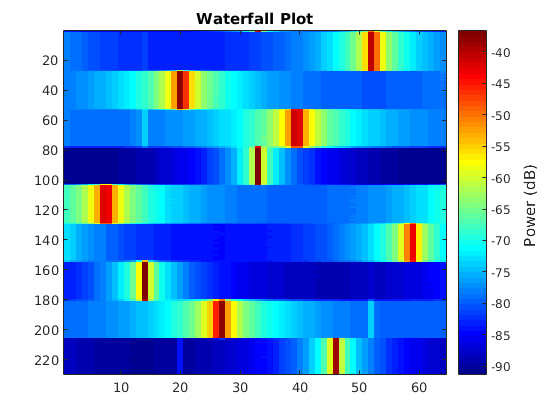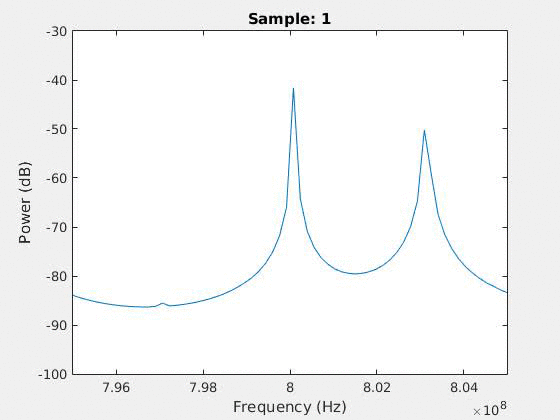Figure 1. Waterfall plot (left) and animated power vs. frequency plot (right) for Experiment 1 generated using wiserd FFT data.

Update: Raw IQ Samples and Processing in MATLAB

Next, using the same frequencies and sampling rate as the original tutorial, we modified the OEDL script in order to collect raw time domain samples using ORBIT as opposed to samples that had already been converted into the frequency domain.

We then wrote a MATLAB script entitled "spectro" which we used to convert the raw data into the frequency domain. From there, we again generate a waterfall plot of the data. See Figure 2.

Using the raw IQ samples instead of preprocessed FFT data allows for more flexibility in our signal processing. We now have access to a much larger collection of data, and we can produce more accurate frequency domain transforms.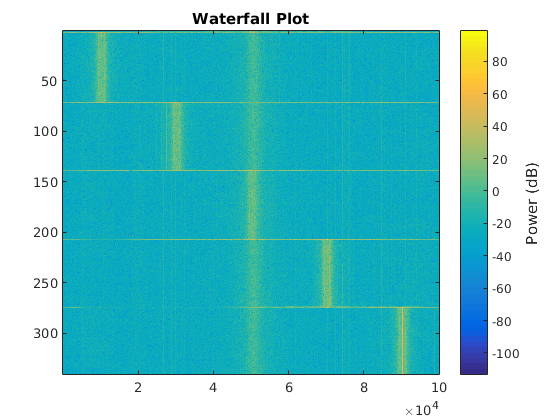Figure 2. Waterfall plot generated from raw IQ samples from Experiment 1.

#### Experiment 2: Signal Transmission and Processing with Two Transmitters and One Receiver

In this experiment, we write an OEDL script that uses two transmitters and one receiver to collect preprocessed FFT samples. Utilizing three USRP2 radios on the grid, two nodes were used as transmitters, and one as a receiver.

The first transmitter starts at a frequency of 798 MHz and increases to 802 MHz, while the second transmitter starts at a frequency of 804 Mhz and increases to 808 MHz all over a span of 2.5 seconds. The receiver has a carrier frequency of 803 MHz and a bandwidth of 12 Mhz.

We then save the FFT data from the receiver to our local device and process in MATLAB, where we generated a waterfall plot of the data. See Figure 3.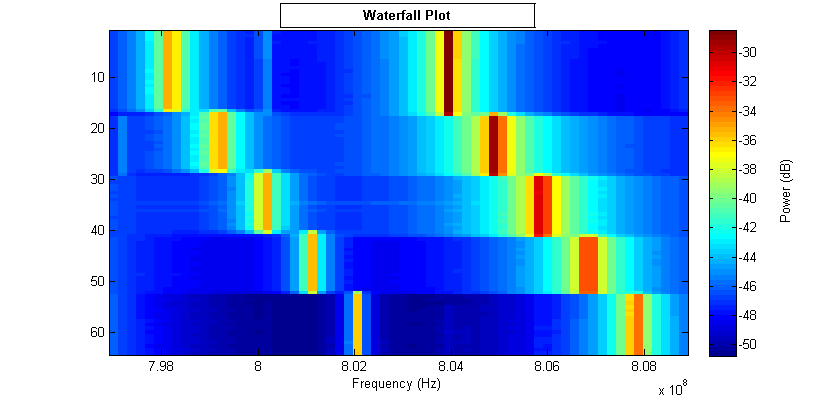Figure 3. Waterfall plot containing frequencies from two transmitters, generated using wiserd FFT data from Experiment 2.

Once we have the experiment set up, we can extract IQ samples from the receiver and use them in our MATLAB processing script.

To reduce the size of our output data, we reduced the receiver sampling rate, shortened the time span of the experiment to 1.5 seconds, and confined the transmitters to a smaller spectrum of frequencies.

The MATLAB script currently generates a waterfall plot, plots individual FFTs and applies a simple peak-finding algorithm to identify possible transmitted frequencies. See Figure 4.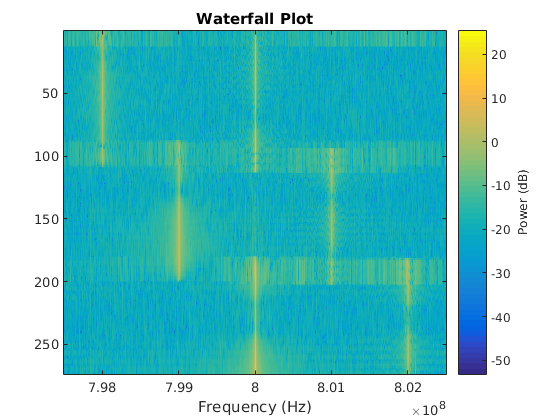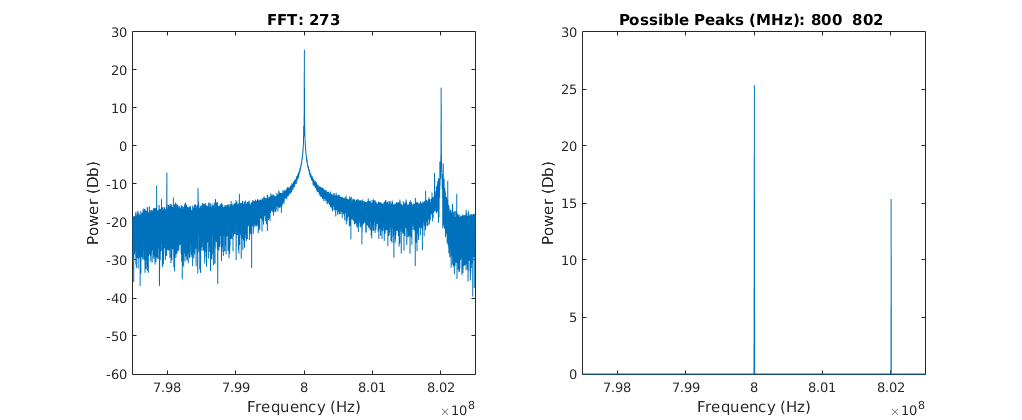Figure 4. Waterfall plot (left) and an example of the peak-finding algorithm applied to an FFT (right), generated using IQ samples from Experiment 2.